# Voltage Regulator 7805

All voltage sources cannot able to give fixed output due to fluctuations in the circuit. For getting constant and steady output, the voltage regulators are implemented. The integrated circuits which are used for the regulation of voltage are termed as voltage regulator ICs. Here, we can discuss the IC 7805.

The voltage regulator IC 7805 is actually a member of the 78xx series of voltage regulator ICs. It is a fixed linear voltage regulator. The xx present in 78xx represents the value of the fixed output voltage that the particular IC provides. For 7805 IC, it is +5V DC regulated power supply. This regulator IC also adds a provision for a heat sink. The input voltage to this voltage regulator can be up to 35V, and this IC can give a constant 5V for any value of input less than or equal to 35V which is the threshold limit.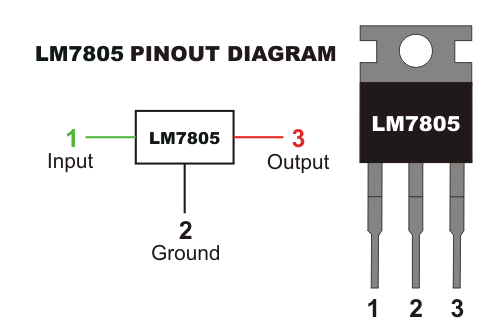PIN 1-INPUT
The function of this pin is to give the input voltage. It should be in the range of 7V to 35V. We apply an unregulated voltage to this pin for regulation. For 7.2V input, the PIN achieves its maximum efficiency.

PIN 2-GROUND
We connect the ground to this pin. For output and input, this pin is equally neutral (0V).

PIN 3-OUTPUT
This pin is used to take the regulated output. It will be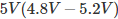## Heat Dissipation in IC 7805

In IC 7805 voltage regulator, lots of energy is exhausted in the form of heat. The difference in the value of input voltage and output voltage comes as heat. So, if the difference between input voltage and the output voltage is high, there will be more heat generation. Without a heat sink, this too much heat will cause malfunction.

We call, the bare minimum tolerable difference between the input and output voltage to keep the output voltage at the proper level as dropout voltage. It is better to keep the input voltage 2 to 3V greater than the output voltage, or a suitable heat sink should be placed to dissipate excess heat. We have to calculate the heat sink size properly. The following formula will give an idea of this calculation.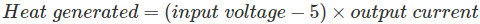Now, we can analyze the relation of generated heat and the input voltage value in this regulator with the following two examples.

Assume a system with input voltage 16V and required output current be 0.5A.
So, heat generated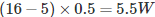Thus, 5.5W heat energy is wasted and the actual energy used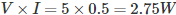That is almost double energy is wasted as heat.
Next, we can consider the case when input is lower, say 9V.
In this case, heat generated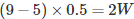From this, we can conclude that for high input voltage, this regulator IC will become highly inefficient. If you’d like to learn more, we have a large range of free digital electronics MCQ questions.

## Internal Block Diagram of 7805 Voltage Regulator

The internal block diagram of IC 7805 is represented in the figure below: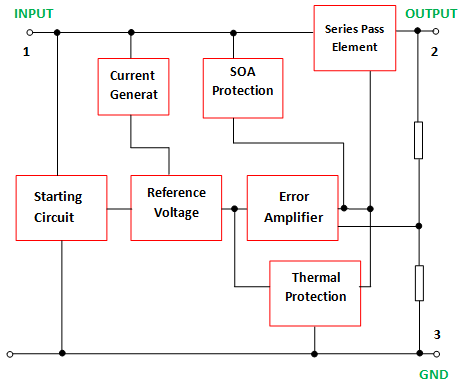The block diagram comprises of an error amplifier, series pass element, current generator, reference voltage, current generator, starting circuit, SOA protection and thermal protection.

Here the operating amplifier performs as an error amplifier. The Zener diode is used for giving the reference voltage. It is shown below.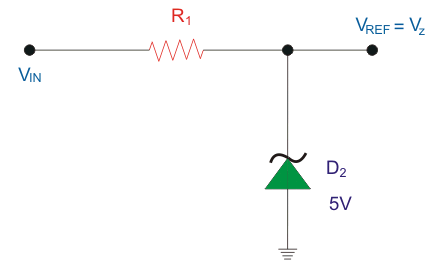Transistor is the series pass element here. It is used for dissipating additional energy in the form of heat. It controls the output voltage by controlling the current among the input and output. SOA is the Safe Operating Area. It is in fact the conditions of voltage and current in which the equipment is expected to work without any self-damage. Here for the SOA protection, bipolar transistor is implemented with a series resistor and an auxiliary transistor. Heat sink is implemented for thermal protection when there is high supply voltage.

## Regulated Power Supply Circuit

The voltage regulator 7805 and the other components are arranged in the circuit as shown in figure.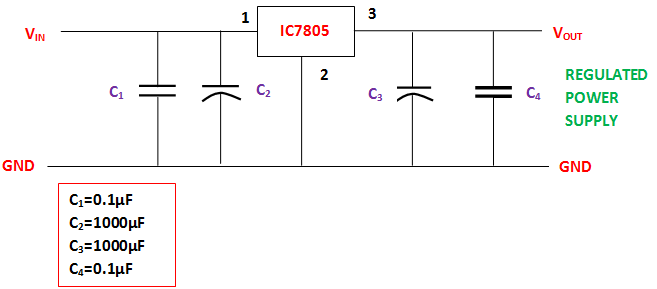The purposes of coupling the components to the IC7805 are explained below.
C1– It is the bypass capacitor, used to bypass very small extent spikes to the earth.
C2 and C3– They are the filter capacitors. C2 is used to make the slow changes in the input voltage given to the circuit to the steady form. C3 is used to make the slow changes in the output voltage from the regulator in the circuit to the steady form. When the value of these capacitors increases, stabilization is enlarged. But these capacitors single-handedly are unable to filter the very minute changes in the input and output voltages.
C4– like C1, it is also a bypass capacitor, used to bypass very small extent spikes to the ground or earth. This is done without influencing other components.

## Applications of Voltage Regulator 7805 IC

• Current regulator
• Regulated dual supply
• Building circuits for Phone charger, UPS power supply circuits, portable CD player etc
• Fixed output regulator